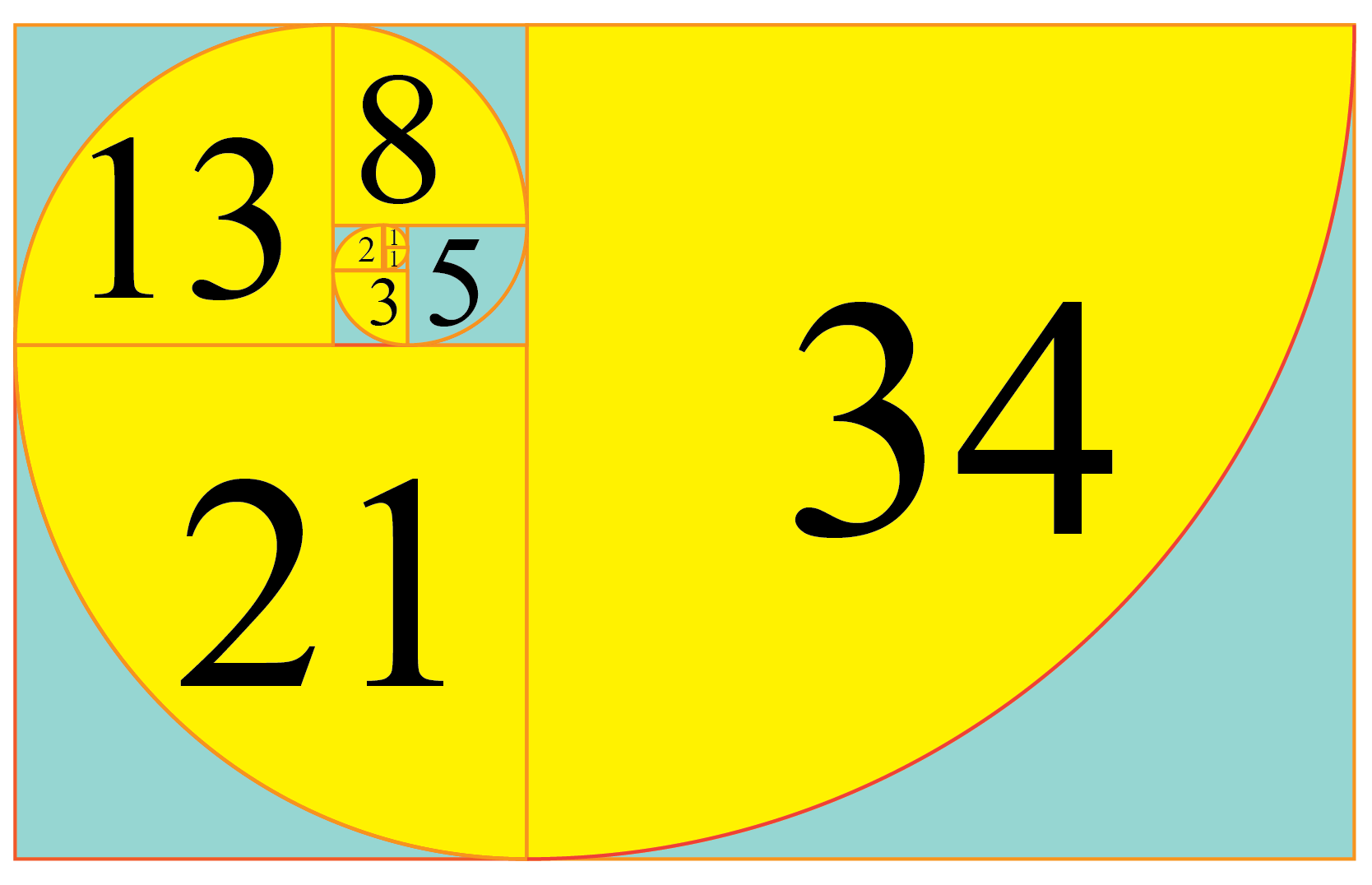# Fibonacci Numbers

Fibonacci Numbers

The Fibonacci sequence was invented by an Italian named Leonardo Pisano Bigollo.

It was an outcome of a mathematical problem in order to keep a check on the pairs of rabbits born every year.

Once you understand the basic concept, can you help us find the first 60 Fibonacci numbres? If you solve this successfully without a Fibonacci number calculator, then you will have grasped the Fibonacci sequence facts, as well as understood the nature of Fibonacci numbers.

Check out the interactive simulations to know more about the lesson and try your hand at solving a few interesting practice questions at the end of the page.

## Lesson Plan

 1 What Are Fibonacci Numbers? 2 Important Notes on Fibonacci Numbers 3 Challenging Questions on Fibonacci Numbers 4 Solved Examples on Fibonacci Numbers 5 Interactives Question on Fibonacci Numbers

## What Are Fibonacci Numbers?

• Fibonacci numbers are a sequence of whole numbers arranged as follows:

0, 1, 1, 2, 3, 5, 8, 13, 21, 34,...

• This sequence is called the Fibonacci sequence.
• Each number in the Fibonacci sequence is given as Fn
• It is an infinite sequence.
• It is represented as the spiral below.Did you know:

• Fibonacci numbers in nature can be shown by an example of petals of a sunflower. They follow the Fibonacci sequence.More Important Topics
Numbers
Algebra
Geometry
Measurement
Money
Data
Trigonometry
Calculus